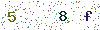﻿ The concepts of lamp beads efficiency - LISUNLISUN

# The concepts of lamp beads efficiency

For lamp beads, there are two concepts of efficiency:

1. Lamp beads luminous efficiency, how much light can be issued every 1W input power. This efficiency is the indicator to measure the light efficiency of lamp beads, not the indicator to measure the light efficiency of total luminaries. Therefore, the light efficiency of single LED is the basic for the light efficiency of total luminaries; it is not equal to the light efficiency of the total luminaries.
2. Lamp beads conversion efficiency means that every 1W input power, how much power is used to emit light. Luminous efficiency is generally used. Conversion efficiency is often used to assess the development level of the chip.

Secondly, the efficiency of lamp beads in the application environment generally does not use. However, it is generally used to assess the quality of number of lamp beads, which is irrespective of the case without driver, the ratio of lamp beads direct consumed power in lamp board and the lamp board total luminous amount. The lumen amount calculated by this way can reflect the average luminous efficiency of a number of lamp beads, to determine the quality of number of lamp beads.

In addition, more people may be familiar with driver efficiency, it is the driver conversion efficiency that is the ratio of drive input power and drive output power.

Finally is the total luminaries luminous efficiency. This formula is simple; it is the ratio of luminaries luminous total amount and input total power. For the optical conversion efficiency generated by the lamp structure is a little special, lamp structure optical conversion efficiency=total luminaries total flux/lamp board total flux, which is defined as the ratio of the light emitted by lamp board of the light emitted by the total luminaries; this contains at least the optical attenuation because of expanding light board or translucent panel.